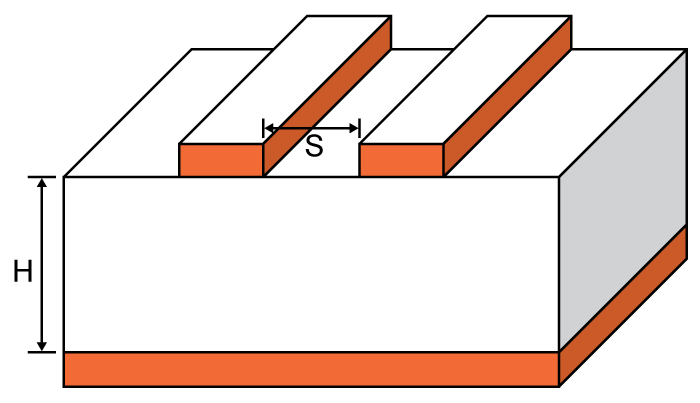# Microstrip Crosstalk Calculator

## This tool is designed to calculate cross-talk coefficient and coupled voltage for a microstrip.

ns
Volts

dB
Volts

### Overview

Crosstalk occurs when a signal "jumps" from one trace to another, a phenomenon that is unintentional and often unwanted. This calculator helps you determine the magnitude of the crosstalk that could occur on a microstrip given its dimensions and source voltage. This tool will also give you the magnitude of the coupled voltage between adjacent traces.### Equations

$$T_{RT} = 1.017\sqrt{\varepsilon _{r\cdot 0.475+0.67}}\cdot L\cdot 2$$

if,

$$\frac{T_{RT}}{T_{R}}\leq 1$$

then,

$$CT_{dB} = 20\log \left ( \frac{1}{1+\left ( \frac{S}{H} \right )^{2}}\cdot \frac{T_{RT}}{T_{R}} \right )$$

$$V_{crosstalk} = V\cdot \frac{1}{1+\left ( \frac{S}{H} \right )^{2}}\cdot \frac{T_{RT}}{T_{R}}$$

else,

$$CT_{dB} = 20\log \left ( \frac{1}{1+\left ( \frac{S}{H} \right )^{2}}\right )$$

$$V_{crosstalk} = V\cdot \frac{1}{1+\left ( \frac{S}{H} \right )^{2}}$$

### Applications

The need for smaller size microwave integrated circuits has led to the development of the microstrip transmission line. Microstrip technology is primarily used because signals, at relatively high frequencies, cannot be routed and interfaced with components by using conventional printed circuit boards.

Crosstalk is the coupling of undesired signals between nearby lines. When designing system interconnections, crosstalk must be taken into consideration. The magnitude of crosstalk can become significantly large and may result in false switching of the digital circuits. It is desirable to predict the behavior of the waves that propagate along the line and to estimate the amount of crosstalk present. The predicted result can be used to determine, in advance, the severity of the interconnection noise in the circuit. With this knowledge, the engineer can anticipate the dynamic behavior of the interconnection line and correct the layout problems and printed circuit boards interconnect accordingly.